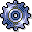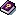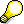Standard Cost EstimatesUse

You usually create a standard cost estimate for a material at the beginning of a fiscal year or a new season. The standard cost estimate is then valid for the entire year or season. You can use it to determine a standard price for materials in this period.

You should not change the standard cost estimate during this period. The results of the cost estimate then remain constant and are not influenced by price fluctuations or changes in the production setup during the course of the planning period.

You valuate the planned quantity structure of a standard cost estimate with standard prices. A standard cost estimate for a material is not linked to an order or to a production version.

Prerequisites

You create a cost estimate based on a costing variant. For standard cost estimates, the costing variant contains the following settings:

• The costing type specifies that the costing results can be updated as the standard price in the material master.
• The valuation variant specifies that the materials are valuated with standard prices or planned prices.

For more information, seePreparing for Costing: Customizing.

For standard cost estimates for materials involving repetitive manufacturing, you must do the following in the MRP view of the material master:

• Set the Repetitive mfg indicator
• Enter a repetitive manufacturing profile

Features

The standard cost estimate calculates a standard price for materials with "S" price control:

• If youmark the standard cost estimate, the system writes the results of the cost estimate into the costing view of the material master record as the future standard price. You can use this price to valuate a material component in the cost estimate.
• If yourelease the standard cost estimate, the system transfers the result of the standard cost estimate into the material master record of the material as the standard price. This price is then active for Financial Accounting and is used for valuation of the material until the next time a standard cost estimate is released.
• From this period on, all transactions involving products produced in-house are valuated in the Logistics module using the standard price (that is, the results of the standard cost estimate). If a material with standard price control is delivered to stock, for example, inventories of this material are valuated with the standard price as determined by the standard cost estimate. This provisional valuation can be corrected at a later date following the settlement of the actual costs that occurred in the period.Valuation using standard prices calculated in the standard cost estimate applies only to materials with "S" price control.

You can also use the results of the standard cost estimate to determine the following data for each production order or run schedule header (make-to-stock production) at the end of the accounting period:

• Variances for the actual costs of a product
• Prices for confirmed scrap quantities
• Target costs for valuating the work in process based on the confirmed quantities

For more information about using thecosting results, see the following documents: